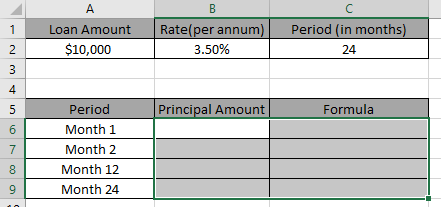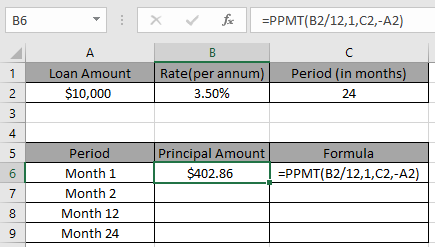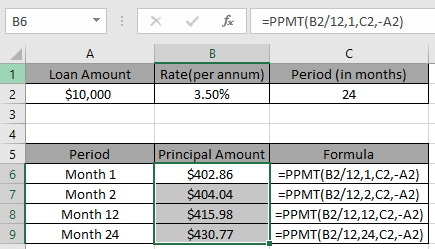# How to use the PPMT function in excel

In this article, we will learn how to use the PPMT function in Excel.

You must be thinking when taking out a loan from a bank how much Principal amount you owe to the bank every month. You can calculate the amount using Excel PPMT function.

Excel PPMT function returns the amount of interest portion for a specific period.
Syntax:

=PPMT (rate, per, nper, pv, [fv], [type])

Rate: Interest rate per period
Per : payment period of interest.
nper : total no. of the payment period.
pv : present value or the total loan amount.
fv [optional] : Extra cash balance if required. Default to 0.
type [optional] : When payments are due. Default is 0

Let’s understand this function using in an example.
Here we have a data set and to get PPMT amount.We need to find the principal amount for 4 different months mentioned in the Period column.

Use this formula to get the principal amount for the first month:

=IPMT( B2/12 , 1 , C2 , -A2 )

Explanation:
B2/12: rate is divided by 12 as we are calculating interest for monthly periods.
1 : per is 1 as we are calculating for the first month. If you wish to find for any specific period. Mention the number for the period you wish to find the interest amount
C2 : total period to pay the amountHere the argument to the function is given as cell reference.
Now we will change the per value to find the principal amount for the specific period.Excel PPMT function does your calculation work. You just have to input numbers and use the right formula.
Hope you understood how to use the PPMT function in Excel. Explore more articles on Excel's financial function here. Please feel free to state your query or feedback for the above article.

Related Articles

How to Use RATE Function in Excel

How to Use IRR function in Excel

How to use the Excel IPMT Function

How to use the Excel CUMIPMT Function

Popular Articles

Edit a dropdown list

If with conditional formatting

If with wildcards

Vlookup by date

Terms and Conditions of use

The applications/code on this site are distributed as is and without warranties or liability. In no event shall the owner of the copyrights, or the authors of the applications/code be liable for any loss of profit, any problems or any damage resulting from the use or evaluation of the applications/code.